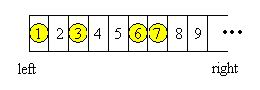# POJ 1704 -Georgia and Bob(ps:看了一些NIM博弈,感觉只有一开始就处于必胜状态的人,才有所谓的最优策略,此最优策略将带领他胜利,而注定失败的人无论如何挣扎,都将走向失败,哇好残酷啊

code:

``````#include <algorithm>
#include <cmath>
#include <cstdio>
#include <cstring>
#include <iostream>
#include <map>
#include <string>
#include <vector>

using namespace std;

typedef long long ll;
typedef unsigned long long ull;

int A;
int main() {
int T;
cin >> T;
while (T--) {
int n;
cin >> n;
for (int i = 0; i < n; ++i) {
cin >> A[i];
}
if (n & 1) A[n++] = 0;
sort(A, A + n);
int x = 0;
for (int i = 0; i + 1 < n; i += 2) {
x ^= (A[i + 1] - A[i] - 1);
}
if (x)
cout << "Georgia will win" << endl;
else
cout << "Bob will win" << endl;
}

return 0;
}
``````